MUSIC AlgorithmNext: MATLAB Simulations Up: Performance Evaluation and Real-Time Previous: NDFE Algorithm

# MUSIC Algorithm

MUSIC detects frequencies in a signal by performing an eigen decomposition on the covariance matrix of a data vector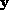of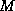samples obtained from the samples of the received signal. The key to MUSIC is its data model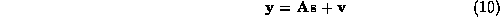whereis a vector ofnoise samples,is a vector of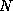signal amplitudes (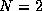for DTMF tones), and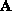is the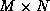Vandermonde matrix of samples of the signal frequencies. If we assume a zero-mean signal and white noise, then the covariance ofhas the form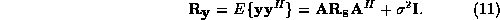Here,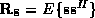is the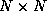signal autocorrelation matrix,is the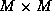identity matrix, and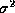is the noise variance. From the eigen decomposition of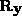, we use the eigenvectors associated with themaximum eigenvalues to define the signal subspace (the column space of), and use the other eigenvectors to define the noise subspace,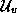. From the orthogonality of the signal and noise subspaces, finding the peaks in the estimator function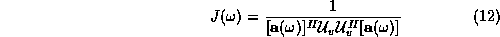for variousvalues yields the strongest frequencies , where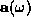refers to the columns of.

MUSIC assumes that the number of samplesand the number of frequenciesare known. The efficiency of MUSIC is the ratio of the theoretical smallest variance, given by the Cramer-Rao Lower Bound (CRLB) , to the variance of the MUSIC estimator: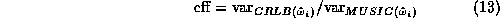The efficiency does not depend on the total number of samples,, (Figure 1) but does depend on(Figure 2). Asincreases, efficiency and computation time increase. We pick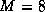, because larger values do not significantly improve the efficiency.

Brian L. Evans, 211-105 Cory Hall, Berkeley, CA 94720-1772